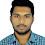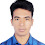# Estimation of Building Materials in Excel Sheet

In this excel sheet, you can estimate building materials in easy ways. You get this excel sheet free. Download this link below.

Here you get,
Estimation of Reinforcement for  Pile,
Estimation of Concrete for  Pile,
Estimation of Reinforcement for  Slab,
Estimation of Reinforcement for  Beam

## Estimation of Building Materials in Excel Sheet

For Example:

### Estimation of Reinforcement for  Pile

For 18" Dia Pile of 45ft, Length

Number of Bar = 7
length of Bar including 2' lapping+2' excess over cut off = 53 Ft
Crossectional area of  16MM Bar= 0.31 in²
Amount of 16MM ber= 178.1057639 Kg
For 18" Dia Pile of 50ft Length.

Number of Bar = 7
length of Bar including 2' lapping+2' excess over cut off = 58 Ft
Crossectional area of  16MM Bar= 0.31 in²
Amount of 16MM ber= 194.9081944 Kg
For 20" Dia Pile of 45ft Length.

Number of Bar = 8
length of Bar including 2' lapping+2' excess over cut off = 53 Ft
Crossectional area of  16MM Bar= 0.31 in²
Amount of 16MM ber= 203.5494444 Kg.
For 20" Dia Pile of 50ft Length.

Number of Bar  = 8
length of Bar including 2' lapping+2' excess over cut off = 58 ft.
Crossectional area of  16MM Bar = 0.31 in²

Amount of 16 mm ber = 222.7522222 Kg.
Number of 18" Dia Pile of 45 ft length= 18.
Number of 18" Dia Pile of 50 ft length= 8.
Number of 20" Dia Pile of 45 ft length= 15.
Number of 20" Dia Pile of 50 ft length= 3.

Total Amount of 16 mm bar for Pile= 8486.667639 Kg.

See all Estimation in this Excel Sheet. thank you.

1.1.